• Python还有很多数据可视化库，本文我将简单介绍12款常用的Python数据可视化库，并在文末送出一本数据可视化书籍！ Python有很多数据可视化库，这些数据可视化库主要分为探索式可视化库和交互式可视化库。前者透过...
大家普遍第一次接触到的Python数据可视化库基本上都是Matplotlib。Python还有很多数据可视化库，本文我将简单介绍12款常用的Python数据可视化库，并在文末送出一本数据可视化书籍！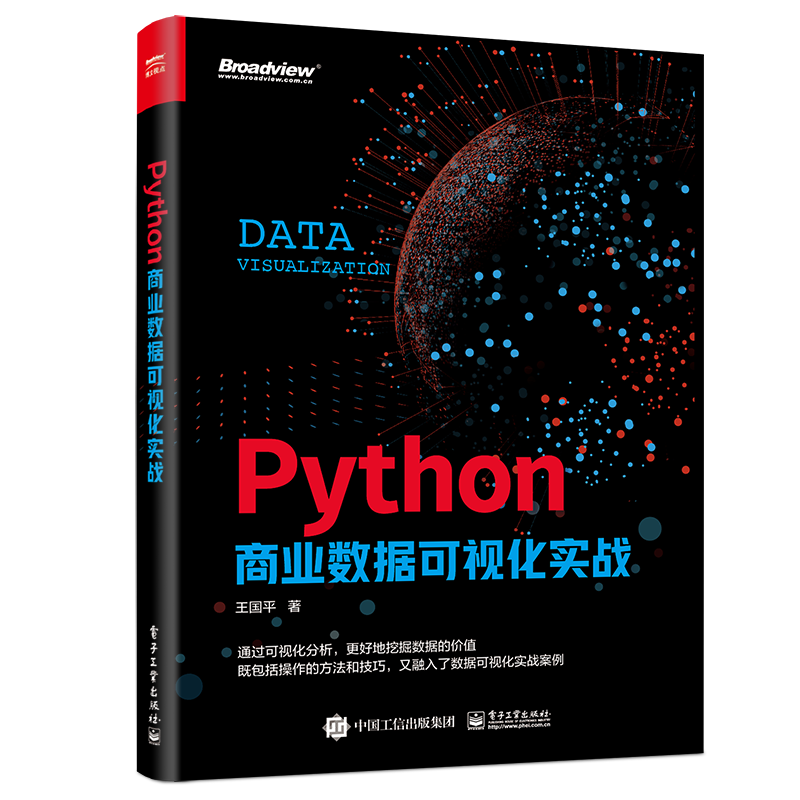Python有很多数据可视化库，这些数据可视化库主要分为探索式可视化库和交互式可视化库。前者透过简单直接的视觉图形，更方便用户看懂原数据，后者主要用于与业务结合过程中展现总体分析结果。

探索式可视化库

探索式分析最大的优势在于，可以让业务人员在海量数据中“自由发挥”，不受数据模型的限制。通过探索式分析和可视化，业务人员可以快速发现业务中存在的问题。

Python探索式可视化库主要包括如下几个。

01 Matplotlib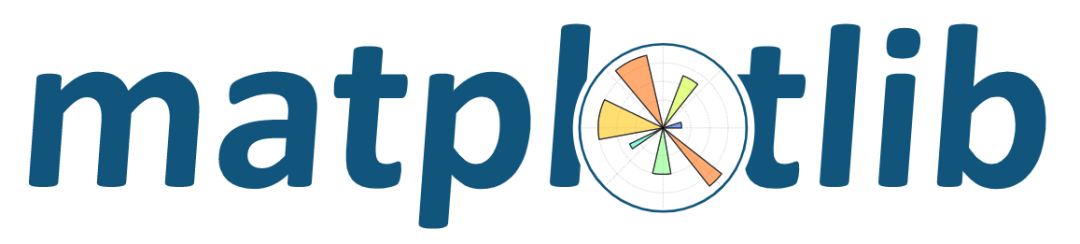Matplotlib是Python数据可视化库的元老，尽管它已有十多年的历史，但仍然是Python社区中使用最广泛的绘图库，编写几行代码即可生成线图、直方图、功率谱密度图、条形图、错误图、散点图等。

Python的可视化库众多，各有各的特点，但是Matplotlib是一个非常基础的Python可视化库，如果需要学习Python数据可视化，那么Matplotlib是非学不可的，之后再学习其他库就比较简单了。Matplotlib的中文学习资料比较丰富，其中最好的学习资料是其官方网站的帮助文档，大家可以在上面查阅自己感兴趣的内容。

02 Seaborn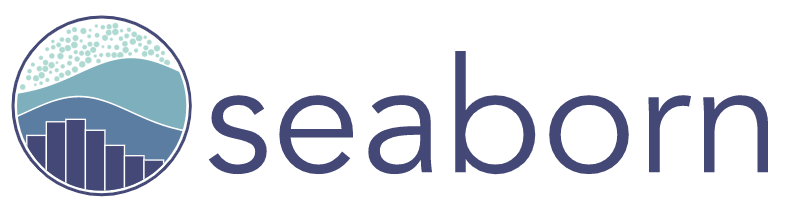Seaborn利用Matplotlib的强大功能，只用几行代码就能创建出漂亮的图表。它们的关键区别在于，Seaborn的默认款式和调色板设计更加美观和现代。由于Seaborn是在Matplotlib基础上构建的，因此用户还需要了解Matplotlib以便调整Seaborn的默认值。Seaborn同Matplotlib一样，也是Python进行数据可视化分析的重要第三方包。Seaborn在Matplotlib的基础上进行了更高级的API封装，使用户绘图更加容易，所绘图形更加漂亮。Seaborn是基于Matplotlib的一个模块，专用于统计可视化，可以和Pandas进行无缝连接，使可视化的初学者更容易上手。相对于Matplotlib，Seaborn语法更简洁，两者的关系类似于Numpy和Pandas的关系。但是需要注意的是，应该把Seaborn视为Matplotlib的补充，而不是替代物。

Seaborn库旨在以数据可视化为中心来挖掘与理解数据，它提供的面向数据集制图函数主要是对行列索引和数组的操作，包含对整个数据集进行内部的语义映射与统计整合，以此生成信息丰富的图表。

03 Pyecharts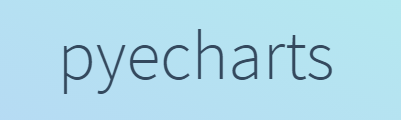Pyecharts是我国开发人员开发的，相比较Matplotlib、Seaborn等可视化库，Pyecharts十分符合国内用户的使用习惯。Pyecharts的目的是实现Echarts与Python的对接，以便在Python中使用Echarts生成图表。

Echarts是Apache软件基金会的一个数据可视化JavaScript库，生成的图的可视化效果非常好，其凭借良好的交互性，精巧的图表设计，得到了众多开发者的认可

04 Missingno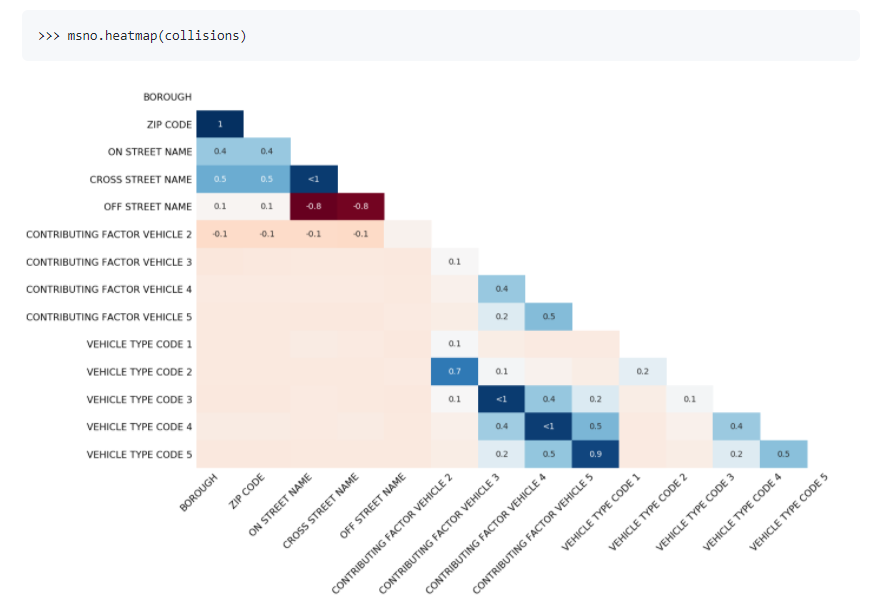处理缺失的数据是一件让人痛苦的事，Missingno通过使用视觉摘要来快速评估数据集的完整性，而不是通过大篇幅的表格。它可以根据热力图或树状图的完成度或点的相关度对数据进行过滤和排序。

交互式可视化库

数据可视化可以是静态的也可以是交互的，交互式的数据可视化是指人们使用计算机和移动设备深入图表和图形的具体细节，然后用交互的方式改变他们看到的数据。Python交互式可视化库主要包括如下几个。

05 Bokeh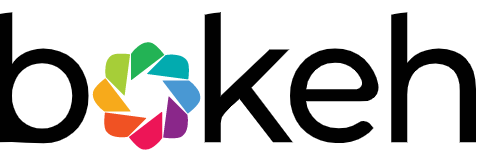Bokeh基于JavaScript实现交互式可视化，它是原生Python语法，它可以在Web浏览器中实现美观的视觉效果。它的优势在于能够创建交互式的网站图，可以很容易地将数据输出为JSON对象、HTML文档或交互式Web应用程序。Bokeh还支持流媒体和实时数据。

但是它也有明显的缺点：一是版本时常更新，最重要的是有时语法不向下兼容，这对于开发者来说是噩梦；二是语法晦涩，与Matplotlib相比，可以说是有过之而无不及。

06 HoloViewsHoloViews是一个开源的Python库，旨在使数据分析和可视化更加简便，可以用非常少的代码行完成数据分析和可视化。

除了默认的Matplotlib后端，它还添加了一个Bokeh后端。结合Bokeh提供的交互式小部件，可以使用HTML5和WebGL快速生成交互式视图，以及进行高维数据的可视化探索。

07 PlotlyPlotly是一个数据可视化的在线平台，与Bokeh一样，Plotly的强项在于制作交互式视图，但它提供了一些在大多数库中没有的图表，如等高线图、树状图和3D图表。可以在线绘制条形图、散点图、饼图、直方图等多种图形，可以画出很多媲美Tableau的高质量图。

Plotly支持在线编辑图形，支持Python、JavaScript、MATLAB和R等多种语言的API。Plotly生成的所有图表实际上都是由JavaScript产生的，无论是在浏览器还是在Jupyter中，所有的可视化、交互都是基于plotly.js的，它是一个高级的声明性图表库，提供了20多种图表类型，包含3D图表、统计图和SVG地图等。

08 pygal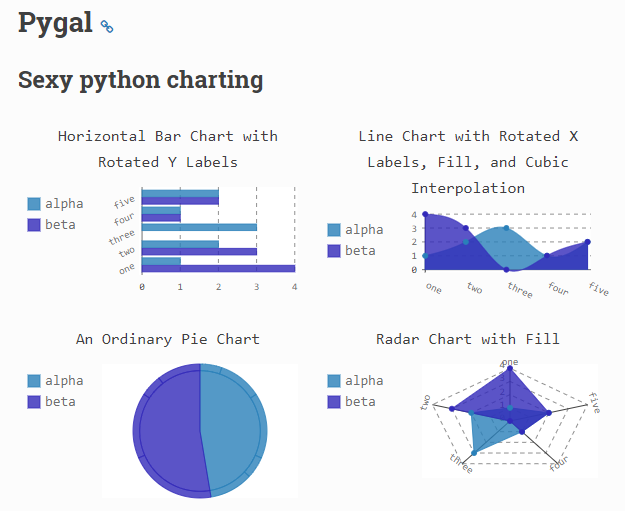与Bokeh和Plotly一样，pygal提供了可以嵌入Web浏览器的交互式视图。区别在于，它能够将图表输出为SVG格式。如果用户使用较小的数据集，则输出位SVG格式的图像就可以了，但是如果用户制作的图表包含数十万个数据点，那么它们就会很难被渲染并变得反应迟钝。

Pygal绘制线图的方法很简单，可以将图表渲染为一个SVG文件，用户使用浏览器打开SVG文件就可以查看生成的图表。

09 plotnineplotnine是Python中图形语法的一种实现，它基于ggplot2包，语法绘图功能强大，可以轻松将数据映射到构成图的可视对象，然后创建自定义的图形。plotnine提供各种不同的可视化视图，易于适应定制化输出，安装十分简单，用户可以通过pip install plotnine命令直接安装。

plotnine的优点为代码简洁，易学；绘制出的图流畅大方；不需要很多的代码就可以绘制出很不错的图。在使用plotnine绘图之前，首先需要理解绘图的基本概念。

10 Altair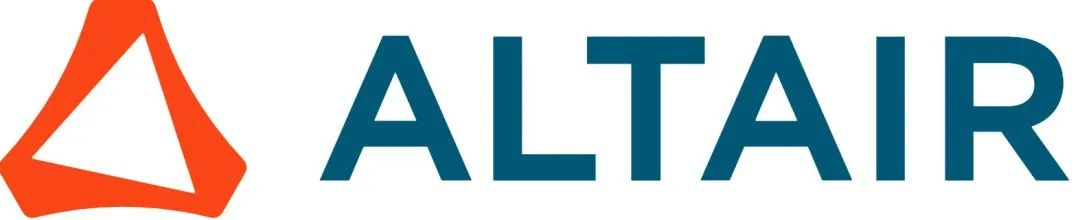Altair是一个专为Python编写，它可以让数据科学家更多地关注数据本身和其内在的联系。因为是基于Vega-Lite（交互式图形语法）的声明性统计可视化库，Altair API具有简单、友好、一致等特点。

声明意味着用户只需要提供数据列与编码通道之间的链接，例如，x轴、y轴、颜色等，其余的绘图细节它会自动处理。声明使Altair变得简单、友好和一致，用户使用Altair可以轻松设计出有效且美观的可视化代码。

11 ggplot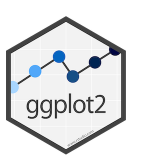ggplot是基于R语言的ggplot2包和Python的绘图系统。ggplot的运行方式与Matplotlib不同，它允许用户对组件进行分层以创建完整的绘图。例如，用户可以从轴开始画，然后添加点，接着添加线、趋势线等。虽然图形语法被认为是绘图的“直观”方法，但经验丰富的Matplotlib用户可能需要时间来适应这个新的方式。

12 Gleam

Gleam的灵感来自R语言的Shiny包。它允许用户仅使用Python脚本就可将分析结果转换为交互式Web应用程序，因此用户不必了解任何其他语言，如HTML、CSS或JavaScript。Gleam适用于任何Python数据可视化库。在创建绘图后，用户可以在它的上面添加字段，以便对数据进行筛选和排序。

展开全文• python可视化学习书籍，中文完整版，可以下载参考，matplotlib
• 上传书籍均经本人鉴定为原版或高清扫描版，且积分一律设置为5积分，更多书籍请查看我的资源。
• 大量的学术期刊，书籍出版物使用它来绘制专业的数据可视化图表。matplotlib 支持跨平台，可运行在 python 脚本，python 解释器, IPython，Jupyter notebook, web 应用服务器，以及四个 GUI（Graphical User ...

matplotlib 是一个 python 的 2D 绘图库。大量的学术期刊，书籍出版物使用它来绘制专业的数据可视化图表。matplotlib 支持跨平台，可运行在 python 脚本，python 解释器, IPython，Jupyter notebook, web 应用服务器，以及四个 GUI（Graphical User Interface） 工具包中。使用matplotlib ，只需要几行代码，便可绘制折线图，直方图，功率谱（power spectra）, 条形图，误差图，散点图等。

1. 安装
pip install matplotlib

2. 导入
ps: 在 jupyter notebook 环境需要添加 %matplotlib inline ，使得绘图生成在 notebook 页面。其他环境需要去掉 %matplotlib inline。
import matplotlib.pyplot as plt
%matplotlib inline

3. 基本概念
matplotlib 中定义了四个基本概念：figure, axes, axis, artist。准确理解这四个基本概念，能更好的理解和熟练使用 matplotlib 绘制你想要的图表。
为了更直观的理解这四个概念，没有比直接使用 matplotlib 将这些抽象的概念绘制出来更好的方式了。
import numpy as np

figure, axes = plt.subplots(1, 2, figsize=(8, 4))
figure.suptitle("figure")

x = np.linspace(0, 2, 100)

axes1, axes2 = axes

axes1.plot(x, x, label='artist legend')
axes2.plot(x, x**2, label='artist legend')

axes1.set_title("axes 1")
axes2.set_title("axes 2")

axes1.set_xlabel("axis x of axes 1")
axes2.set_xlabel("axis x of axes 2")

axes1.set_ylabel("axis y of axes 1")
axes2.set_ylabel("axis y of axes 2")

axes1.legend()
axes2.legend()

axes1.grid()
axes2.grid()

plt.show()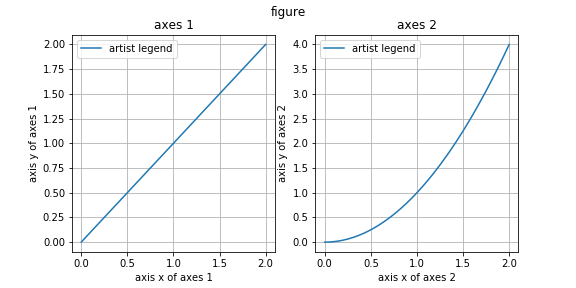通过观察绘制出来的图表，我们可以很直观的看到，各个“抽象概念”的在图表中对应的具体表示，以及它们之间的层次关系。
有了这些可视化呈现，再来逐一理解它们，就很容易了。
3.1 figure
从图中可以看出，figure 表示整个绘图区域，它更多扮演的就是一个容器的角色，所有其他绘图元素都必须要包含在 figure 中，通过 figsize 参数限定 figure 大小，同时也限定了绘图区域的大小。此外，通过使用 figure.suptitle(title) 方法，也可以为整个绘图区域，设置一个标题，但这不是必需的（事实上，如果不给 figure 设置标题，我们这里就无法直观感知到它作为容器的存在）。
由此可见，要使用 matplotlib 绘制图表或任何可视化呈现，就必须先显式或隐式（直接使用 plt.plot 等函数绘制时）创建一个 figure 对象。
下面，简单介绍两种最常用的创建 figure 的方法。根据个人习惯，可任选一种方式即可。个人更多使用第二种方式。
3.1.1 plt.figure(num=None, figsize=None, dpi=None, facecolor=None, edgecolor=None, frameon=True, clear=False)
plt.figure() 是任何创建 figure 对象的底层工厂函数。它提供创建 figure 了所有细节。

参数
描述

num
整数或字符串。figure 编号，即 figure 对象的 number 属性，可以理解为 figure 对象的 ID；如果编号为 num 的 figure 在上下文中已经存在，则直接返回存在 figure 引用，而不创建新的 figure; 默认为 None , 会创建一个新的 figure 对象，并将 number 加1 。

figsize
元组。figure 的宽高，单位 英尺。默认值为 matplotlib.rcParams["figure.figsize"] 。

dpi
整数。分辨率。默认值为 matplotlib.rcParams["figure.dpi"] 。

facecolor
字符串或颜色对象。背景颜色。默认为白色。

edgecolor
字符串或颜色对象。边框颜色。默认为白色。

frameon
Bool。

clear
Bool。为 True 时，清空当前已经存在的 figure 的绘制。默认不清空。

代码示例，理解 num 的作用：
figure1 = plt.figure()
print("this is a new figure instance, whose number is {}.\n".format(figure1.number))
figure2 = plt.figure()
print("this is a new figure instance, whose number is {}.\n".format(figure2.number))

figure1_ref = plt.figure(num=1) # 等效于 plt.figure(1)
print("this is a existed figure instance, whose number is {}.\n".format(figure1_ref.number))
if figure1_ref == figure1:
print("figure1_ref is equal to figure1.")

plt.show()

可以看到，上面脚本运行后，并没有显示可见的图表。正如上文所说，figure 仅仅是个容器，真正我们所想到的包含在 figure 容器中，带有坐标系的一个个图表，是下文将要介绍的 axes 。使用 plt.figure() 方法创建的 figure 要显示图表，必须调用 plt.plot() 等绘制函数。代码如下：
# figure 1
plt.figure(figsize=(9, 3))
plt.suptitle("figure 1")

names = ['a', 'b', 'c']
values = [1, 10, 100]

plt.subplot(131)
plt.bar(names, values)

plt.subplot(132)
plt.scatter(names, values)

plt.subplot(133)
plt.plot(names, values)

plt.show()

# figure 2
plt.figure(figsize=(9, 3))
plt.suptitle("figure 2")

names = ['d', 'e', 'f']
values = [100, 10, 1]

plt.subplot(131)
plt.bar(names, values)

plt.subplot(132)
plt.scatter(names, values)

plt.subplot(133)
plt.plot(names, values)

plt.show()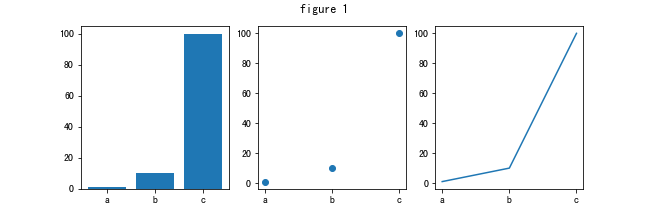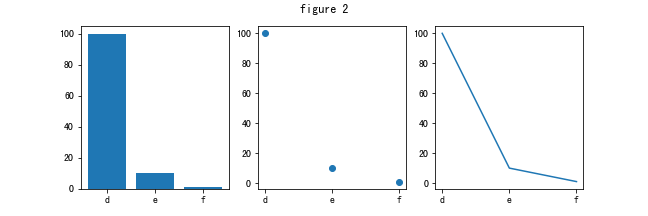3.1.2 plt.subplots(nrows=1, ncols=1, sharex=False,  sharey=False,  figsize=None)
plt.subplots() 是 plt.figure() 的上层封装，提供了一次创建 figure 和 axes 便捷方式。事实上，除了 nrows，ncols，sharex，sharey 等新增的参数，任何 plt.figure() 支持的参数，都可以通过 plt.subplots() 传递, 例如 figsize 参数。
plt.subplots() 它返回一个元组。元组的第一个元素为 figure 对象；第二个元素是用于绘制图表的 axes 对象，需要注意的是，通过参数 nrows，ncols 创建不只一个 axes 时，元组的第二个元素是一个 axes 对象的 numpy 数组。
可见，不同于 plt.figure() ，plt.subplots() 在创建 figure 对象时，同时也创建了一个或多个 axes 对象。因此，创建后就可以看到图表。
如下代码，创建了包含 2 行 3 列，共 6 个 axes 的 figure 对象。
figure, axes = plt.subplots(2, 3, figsize=(9, 6))
figure.suptitle("2 rows 3 cols")
plt.show()

print("type of return tuple is {}".format(type(axes)))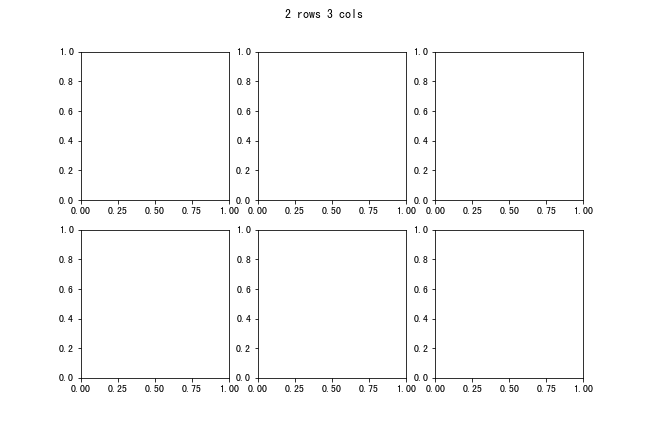如果省略 nrows 和 ncols, 将只创建一个 axes。如下：
figure, axes = plt.subplots()
figure.suptitle("only one axes")
plt.show()

print("type of return tuple is {}".format(type(axes)))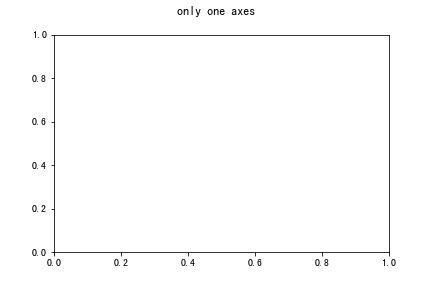3.2 axes

“This is what you think of as ‘a plot’”.

经过上面的论述，相信理解上面这句话，简直 “So easy!”。没错，axes 正是我们绘制各种绚丽图表的真正主角！
上文已经提到，使用 plt.subplots() 方法创建图表，会返回所包含的 axes 对象。这些 axes 对象就是 matplotlib 提供绘制图表的面向对象接口。使用 axes 对象的各种绘制方法，可以在图表中对应 axes 坐标空间，绘制任何图表，文字和图片。
3.2.1 plot([x], y, [fmt])
以 x 为横轴，y 为纵轴，fmt 为格式字符串（定义颜色，标记，线型等），绘制线图或标记。并且可以定义多个 x, y, fmt 组合，一次绘制多个不同图形。
以下提供一些绘制示例。
在同一个 axes 中绘制不同图形 ：
figure, axes = plt.subplots()
t = np.arange(0., 5., 0.2)

axes.plot(t, t, 'r--', t, t**2, 'bs', t, t**3, 'g^')

plt.show()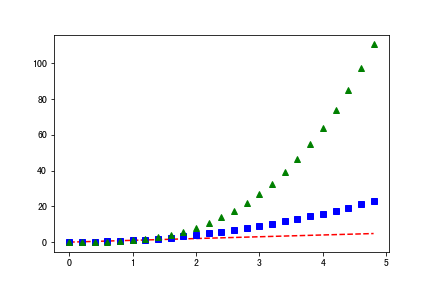在一个 figure 中的两个 axes 绘制不同图形，并且共享 x 轴(axis)：
def f(t):
return np.exp(-t) * np.cos(2*np.pi*t)

t1 = np.arange(0.0, 5.0, 0.1)
t2 = np.arange(0.0, 5.0, 0.02)

figure, axes = plt.subplots(2, 1, sharex=True)
ax1, ax2 = axes

ax1.plot(t1, f(t1), 'bo', t2, f(t2), 'k')
ax2.plot(t2, np.cos(2*np.pi*t2), 'r--')

plt.show()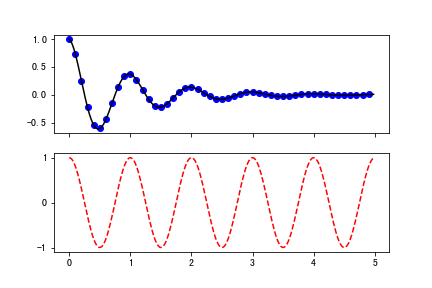3.2.2 散点图: scatter(x, y, s=None, c=None,  alpha=None)
x 横轴；y 纵轴；s 点的大小，默认是 rcParams['lines.markersize'] 的平方；c 颜色；alpha 透明度。
np.random.seed(19680801)

N = 50
x = np.random.rand(N)
y = np.random.rand(N)
colors = np.random.rand(N)
area = (30 * np.random.rand(N))**2

plt.scatter(x, y, s=area, c=colors, alpha=0.5)

plt.show()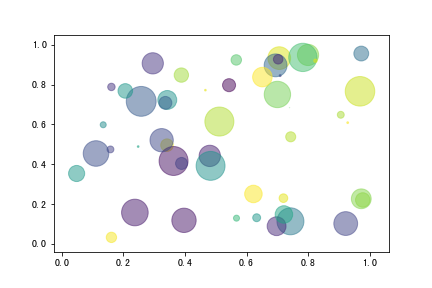3.2.3 条形图: bar(x, height, width=0.8)
x 横坐标位置; height 条形高度; width 条形宽度。
plt.rcParams['font.sans-serif'] = ['SimHei']  # 用来正常显示中文标签
plt.rcParams['axes.unicode_minus'] = False  # 用来正常显示负号

labels = ['G1', 'G2', 'G3', 'G4', 'G5']
men_means = [20, 34, 30, 35, 27]
women_means = [25, 32, 34, 20, 25]

x = np.arange(len(labels))  # 标签位置
width = 0.35  # 条形宽度

fig, ax = plt.subplots()
# 绘制条形图
rects1 = ax.bar(x - width/2, men_means, width, label='男')
rects2 = ax.bar(x + width/2, women_means, width, label='女')

ax.set_ylabel('得分')
ax.set_title('分小组性别得分')
ax.set_xticks(x)
ax.set_xticklabels(labels)
ax.legend()

def autolabel(rects):
"""在每个条形上添加文本，显示条形的高度"""
for rect in rects:
height = rect.get_height()
ax.annotate('{}'.format(height),
xy=(rect.get_x() + rect.get_width() / 2, height),
xytext=(0, 3),  # 增加三个点的垂直偏移
textcoords="offset points",
ha='center', va='bottom')

autolabel(rects1)
autolabel(rects2)

fig.tight_layout()

plt.show()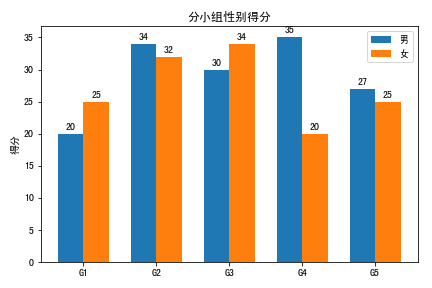3.2.4 水平条形图: barh(y, width, height=0.8)
y 纵坐标位置; width 条形宽度; height 条形高度。
category_names = ['Strongly disagree', 'Disagree',
'Neither agree nor disagree', 'Agree', 'Strongly agree']
results = {
'Question 1': [10, 15, 17, 32, 26],
'Question 2': [26, 22, 29, 10, 13],
'Question 3': [35, 37, 7, 2, 19],
'Question 4': [32, 11, 9, 15, 33],
'Question 5': [21, 29, 5, 5, 40],
'Question 6': [8, 19, 5, 30, 38]
}

def survey(results, category_names):
"""
Parameters
----------
results : dict
A mapping from question labels to a list of answers per category.
It is assumed all lists contain the same number of entries and that
it matches the length of *category_names*.
category_names : list of str
The category labels.
"""
labels = list(results.keys())
data = np.array(list(results.values()))
data_cum = data.cumsum(axis=1)
category_colors = plt.get_cmap('RdYlGn')(
np.linspace(0.15, 0.85, data.shape))

fig, ax = plt.subplots(figsize=(9.2, 5))
ax.invert_yaxis()
ax.xaxis.set_visible(False)
ax.set_xlim(0, np.sum(data, axis=1).max())

for i, (colname, color) in enumerate(zip(category_names, category_colors)):
widths = data[:, i]
starts = data_cum[:, i] - widths
ax.barh(labels, widths, left=starts, height=0.5,
label=colname, color=color)
xcenters = starts + widths / 2

r, g, b, _ = color
text_color = 'white' if r * g * b < 0.5 else 'darkgrey'
for y, (x, c) in enumerate(zip(xcenters, widths)):
ax.text(x, y, str(int(c)), ha='center', va='center',
color=text_color)
ax.legend(ncol=len(category_names), bbox_to_anchor=(0, 1),
loc='lower left', fontsize='small')

return fig, ax

survey(results, category_names)
plt.show()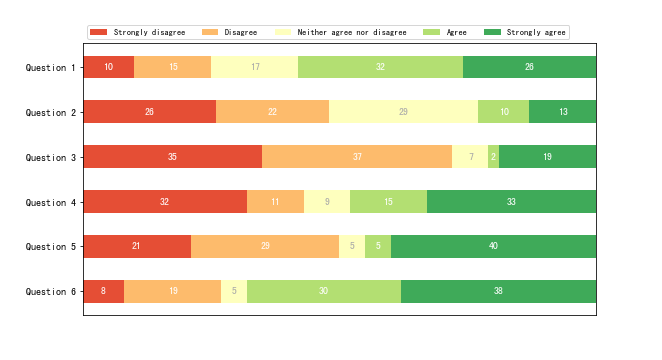3.2.5 饼图: pie(data, explode=None, labels=None, colors=None, autopct=None…)
fig, ax = plt.subplots(figsize=(8, 4), subplot_kw=dict(aspect="equal"))

recipe = ["375 g flour",
"75 g sugar",
"250 g butter",
"300 g berries"]

data = [float(x.split()) for x in recipe]
ingredients = [x.split()[-1] for x in recipe]

def func(pct, allvals):
absolute = int(pct/100.*np.sum(allvals))
return "{:.1f}%\n({:d} g)".format(pct, absolute)

wedges, texts, autotexts = ax.pie(data, autopct=lambda pct: func(pct, data),
textprops=dict(color="w"))

ax.legend(wedges, ingredients,
title="Ingredients",
loc="center left",
bbox_to_anchor=(1, 0, 0.5, 1))

plt.setp(autotexts, size=8, weight="bold")

ax.set_title("Matplotlib bakery: A pie")

plt.show()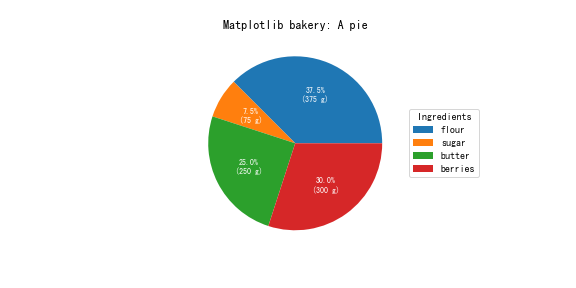3.2.6 直方图 hist(data, bins=None, range=None, density=None, weights=None…)
np.random.seed(19680801)

# 样本数据
mu = 100  # 均值
sigma = 15  # 标准差
x = mu + sigma * np.random.randn(437)

num_bins = 50

fig, ax = plt.subplots()

# 绘制直方图
n, bins, patches = ax.hist(x, num_bins, density=1)

# 最佳拟合线
y = ((1 / (np.sqrt(2 * np.pi) * sigma)) *
np.exp(-0.5 * (1 / sigma * (bins - mu))**2))

ax.plot(bins, y, "--")
ax.set_xlabel("智商")
ax.set_ylabel("概率密度")
ax.set_title("IQ直方图: 平均值=100, 标准差=15")

# 调整间距以防止 ylabel 被遮挡
fig.tight_layout()
plt.show()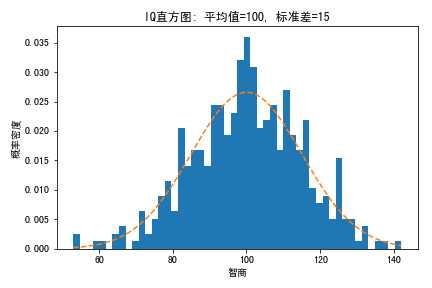3.2.7 2维直方图: hist2d(x, y, bins=10, range=None, density=False, weights=None, …)
import matplotlib.colors as mcolors
from numpy.random import multivariate_normal

data = np.vstack([
multivariate_normal([10, 10], [[3, 2], [2, 3]], size=100000),
multivariate_normal([30, 20], [[2, 3], [1, 3]], size=1000)
])

gammas = [0.8, 0.5, 0.3]

fig, axes = plt.subplots(nrows=2, ncols=2, figsize=(8, 6))

axes[0, 0].set_title('Linear normalization')
axes[0, 0].hist2d(data[:, 0], data[:, 1], bins=100)

for ax, gamma in zip(axes.flat[1:], gammas):
ax.set_title(r'Power law $(\gamma=%1.1f)$' % gamma)
ax.hist2d(data[:, 0], data[:, 1],
bins=100, norm=mcolors.PowerNorm(gamma))

fig.tight_layout()

plt.show()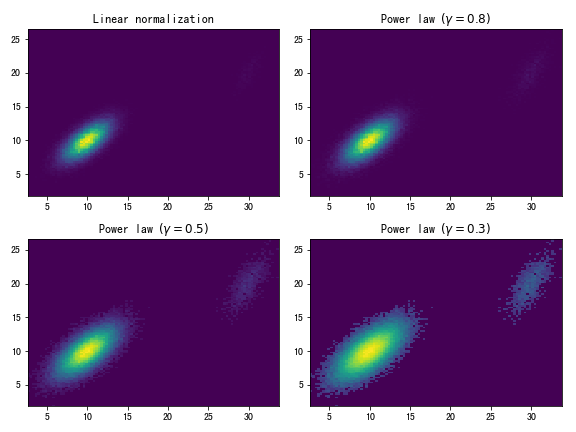3.2.8 显示图片: imshow(X)
with open('dog.jpeg', 'rb') as image_file:
image = plt.imread(image_file)

fig, ax = plt.subplots()
ax.imshow(image)
ax.set_title("Hi, 我们又见面了！")

ax.axis('off')
plt.show()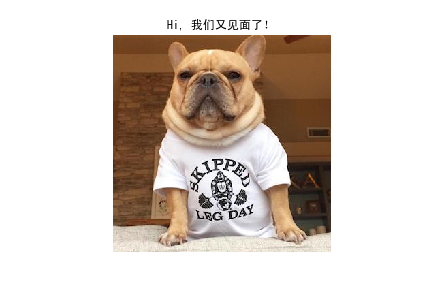3.3 axis
虽然 axis 和 axes 中文翻译都是轴的意思，但是，axes 的意思更像是由 axis 构成的坐标空间，正如上文介绍的，我们可以在这个坐标空间绘制各种图像和显示图片；如果说 axes 是抽象意义的“轴”，那么 axis 就是真正意义的坐标轴；2维绘图中，只有两个 axis 即 横轴 x 和纵轴 y。由于 axis 是构成 axes 一部分，因此我们可以使用 axes 对象的方法，来设置 x 轴和 y 轴的属性。
figure, axes = plt.subplots(1, 2, figsize=(6, 3))

ax1, ax2 = axes

# 设置坐标显示区间
xmin, xmax, ymin, ymax = 0, 10, 50, 100
ax1.axis([xmin, xmax, ymin, ymax])

# 隐藏 axis
ax2.set_axis_off()
ax2.set_title("axis off.")

plt.show()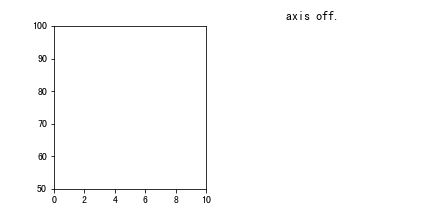3.4 artist
artist 中文翻译是艺术家，在 matplotlib 中，它具有更一般的意义，即所有可见的元素都是 artist。如: title, legend, grid, axis, tick, label, text等等。
np.random.seed(19680801)
data = np.random.randn(2, 100)

fig, axs = plt.subplots(2, 2, figsize=(5, 5))
axs[0, 0].hist(data)
axs[1, 0].scatter(data, data)
axs[0, 1].plot(data, data)
axs[1, 1].hist2d(data, data)

plt.show()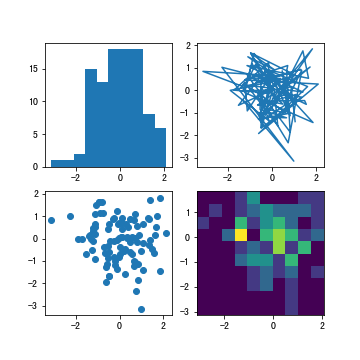猜你喜欢

 python 科学计算的基石 numpy（一）
 python 数据分析工具包 pandas（一）

坚持写专栏不易，如果觉得本文对你有帮助，记得点个赞。感谢支持！

个人网站: https://kenblog.top
github 站点: https://kenblikylee.github.io
掘金: https://juejin.im/user/5bd2b8b25188252a784d19d7
知乎: https://www.zhihu.com/people/kenbliky/posts微信扫描二维码 获取最新技术原创


展开全文matplotlib
• 前言随着互联网的飞速发展，人们在互联网上...在数据分析领域，Python语言简单易用，第三方库强大，并提供了完整的数据分析框架，因此深受数据分析人员的青睐，Python已经当仁不让地成为数据分析人员的一把利器。因...
前言随着互联网的飞速发展，人们在互联网上的行为产生了海量数据，对这些数据存储、处理与分析带动了大数据技术的发展。其中，数据挖掘和分析技术可以帮助人们对庞大的数据进行相关分析，找到有价值的信息和规律，使得人们对世界的认识更快、更便捷。在数据分析领域，Python语言简单易用，第三方库强大，并提供了完整的数据分析框架，因此深受数据分析人员的青睐，Python已经当仁不让地成为数据分析人员的一把利器。因此，本书从Python数据分析的基础知识入手，结合大量的数据分析示例，系统地介绍数据分析和可视化绘图的方法，带领读者一步步掌握Python数据分析的相关知识，提高读者解决实际问题的能力。本书特色(1) 内容全面，讲解系统。(2) 给出了数据分析环境的安装和配置步骤。(3) 详细介绍了使用Python进行数据分析与可视化的方法。(4) 提供了多个有较高应用价值的项目案例，有很强的实用性。(5) 提供丰富的配套资源。本书内容第1章数据分析与可视化概述，主要介绍数据分析与可视化的基本内容，数据、数据分析和数据挖掘的关系，数据分析与可视化的常用工具，Python数据分析与可视化的主要库以及Jupyter Notebook的基本使用方法。第2章Python编程基础，主要介绍Python语言的基本语法、内建数据结构、函数以及文件操作。第3章NumPy数值计算基础，主要介绍数组及其索引、数组运算、数组读/写及常用的统计与分析方法。第4章Pandas统计分析基础，主要介绍Pandas数据结构、索引操作、数据运算、分组汇总聚合、透视表以及Pandas的常用绘图。第5章Pandas数据载入与预处理，主要针对数据预处理阶段的需求，介绍使用Pandas载入数据、合并数据、数据清洗、数据标准化及数据转换的典型方法。第6章Matplotlib数据可视化基础，主要介绍Pyplot绘图的基本语法、常用参数，各类常用图形的绘制及词云的简单用法。第7章Seaborn可视化，主要介绍Seaborn可视化中的风格与主题设置及常见绘图的基本用法。第8章pyecharts可视化，主要介绍pyecharts的安装与导入、绘图主要过程以及柱状图、饼图、漏斗图、散点图、K线图、仪表盘、词云、地图及组合图表的绘制方法。第9章时间序列数据分析，主要介绍时间序列数据分析的基本方法，包括Pandas中的日期型数据、日期的范围、频率及日期的操作。第10章SciPy科学计算，主要介绍SciPy中的常数和特殊函数、线性代数运算、优化、稀疏矩阵处理及简单的图像处理等内容。第11章统计与机器学习，主要介绍sklearn库的基本功能、典型分类、聚类算法以及主成分分析方法及应用。第12章图像数据分析，主要介绍OpenCV的导入、图像的基本操作、SIFT和SURF特征点的提取及图像的降噪。第13章综合案例，介绍两个综合案例，针对职业人群体检数据和股票数据，结合前面章节介绍的数据分析和数据可视化技术，实现数据分析与可视化。本书配套资源 教学大纲、教学课件、电子教案、程序源码、教学进度表，扫描封底的课件二维码可以下载。 420分钟的视频讲解，扫描书中相应位置的二维码可以在线观看、学习。本书由魏伟一、李晓红编写。由于编者水平有限，书中难免存在疏漏和不足之处，敬请读者批评指正。编者2019年10月more >
展开全文• 第一篇文章里已经通过写python爬虫程序获取到了豆瓣电影Top250有关的数据，这里对保存在Excel里的电影信息进行数据分析和可视化。 很多人学习python，不知道从何学起。 很多人学习python，掌握了基本语法过后，不...
第一篇文章里已经通过写python爬虫程序获取到了豆瓣电影Top250有关的数据，这里对保存在Excel里的电影信息进行数据分析和可视化。

很多人学习python，不知道从何学起。
很多人学习python，掌握了基本语法过后，不知道在哪里寻找案例上手。
很多已经做案例的人，却不知道如何去学习更加高深的知识。
那么针对这三类人，我给大家提供一个好的学习平台，免费领取视频教程，电子书籍，以及课程的源代码！
QQ群：101677771

技术要点

pandas读取Excel中指定列的数据
collections.Counter用来统计相关元素出现的次数
matplotlib绘制条形图、散点图
wordcloud绘制词云
1. 上映高分电影数量最多的年份Top10

import collections
import pandas as pd
from matplotlib import pyplot as plt

# 读取数据
df = pd.read_excel("movie.xlsx")
# print(type(df))    # <class 'pandas.core.frame.DataFrame'>

show_time = list(df["上映时间"])
# 有上映时间数据是1961(中国大陆)这样的  处理一下  字符串切片
show_time = [s[:4] for s in show_time]

show_time_count = collections.Counter(show_time)

# 取数量最多的前10  得到一个列表  里面每个元素是元组
# (年份, 数量)
show_time_count = show_time_count.most_common(10)
# 字典推导式
show_time_dic = {k: v for k, v in show_time_count}

# 按年份排序
show_time = sorted(show_time_dic)
# 年份对应高分电影数量
counts = [show_time_dic[k] for k in show_time]

plt.figure(figsize=(9, 6), dpi=100)
# 设置字体
plt.rcParams['font.sans-serif'] = ['Microsoft YaHei']

# 绘制条形图
plt.bar(show_time, counts, width=0.5, color="cyan")

# y轴刻度重新设置一下
plt.yticks(range(0, 16, 2))

# 添加描述信息
plt.xlabel("年份")
plt.ylabel("高分电影数量")
plt.title("上映高分电影数量最多的年份Top10", fontsize=15)

# 添加网格  网格的透明度  线条样式
plt.grid(alpha=0.2, linestyle=":")

plt.show()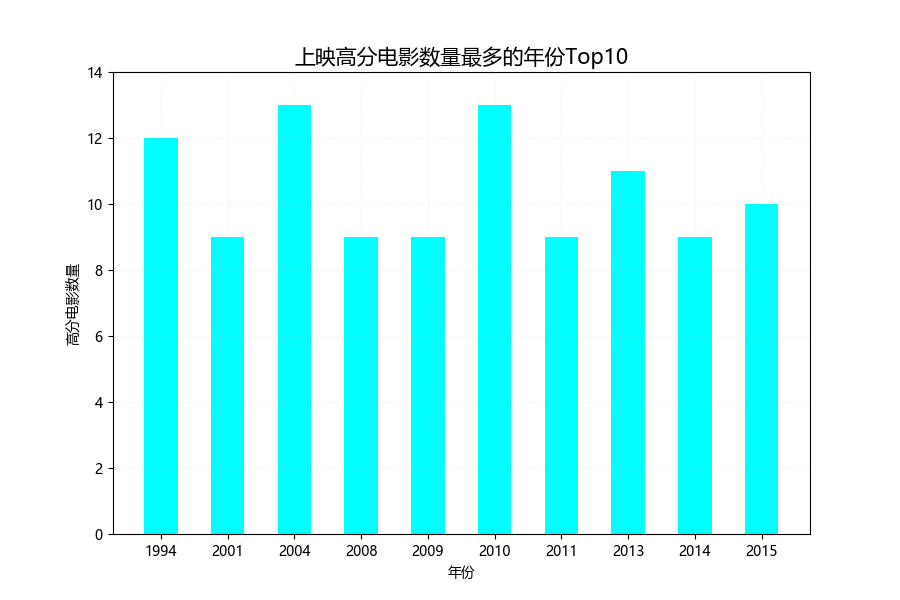2. 豆瓣电影Top250评分-排名的散点分布

import pandas as pd
from matplotlib import pyplot as plt

# 读取数据
df = pd.read_excel("movie.xlsx")

# 豆瓣电影Top250  排名  评分  散点图   描述关系
rating = list(df["排名"])
rating_score = list(df["评分"])

plt.figure(figsize=(9, 6), dpi=100)
# 设置字体
plt.rcParams['font.sans-serif'] = ['Microsoft YaHei']

# 绘制散点图  设置点的颜色
plt.scatter(rating_score, rating, c='r')

# 添加描述信息  设置字体大小
plt.xlabel("评分", fontsize=12)
plt.ylabel("排名", fontsize=12)
plt.title("豆瓣电影Top250评分-排名的散点分布", fontsize=15)

# 添加网格  网格的透明度  线条样式
plt.grid(alpha=0.5, linestyle=":")

plt.savefig('test2.PNG')
plt.show()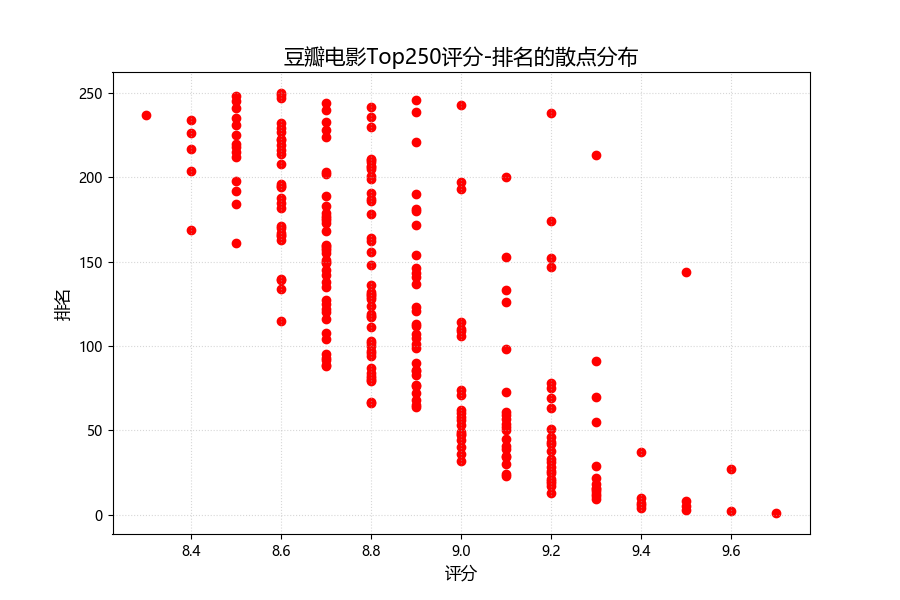3. 电影类型分析

import collections
import xlrd
import matplotlib.pyplot as plt
from wordcloud import WordCloud

# 读取数据
data = xlrd.open_workbook('movie.xlsx')
table = data.sheets()
type_list = []
for i in range(1, table.nrows):
x = table.row_values(i)
genres = x.split(" ")
for j in genres:
type_list.append(j)

type_count = collections.Counter(type_list)

# 绘制词云
my_wordcloud = WordCloud(
max_words=100,           # 设置最大显示的词数
font_path='simhei.ttf',  # 设置字体格式
max_font_size=66,        # 设置字体最大值
random_state=30,         # 设置随机生成状态，即多少种配色方案
min_font_size=12,        # 设置字体最小值
).generate_from_frequencies(type_count)

# 显示生成的词云图片
plt.imshow(my_wordcloud, interpolation='bilinear')
plt.axis('off')
plt.savefig('test3.PNG')
plt.show()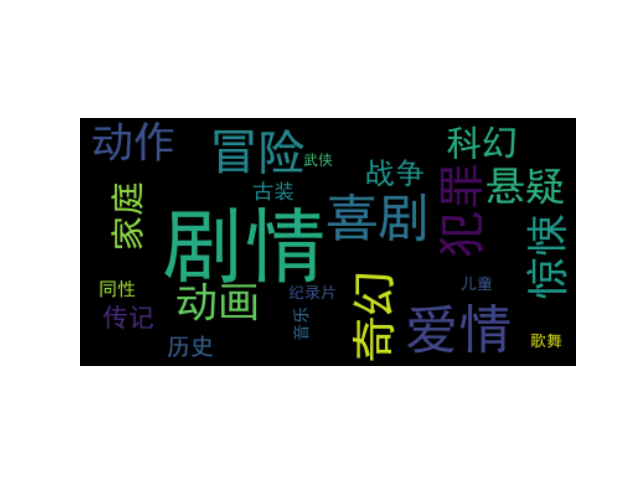4. 国家或地区上榜电影数量最多的Top10

import pandas as pd
import collections
from matplotlib import pyplot as plt

df = pd.read_excel('movie.xlsx')
area = list(df['上映地区'])
sum_area = []
for x in area:
x = x.split(" ")
for i in x:
sum_area.append(i)

area_count = collections.Counter(sum_area)
area_dic = dict(area_count)
area_count = [(k, v) for k, v in list(area_dic.items())]
# 按国家或地区上榜电影数量排序
area_count.sort(key=lambda k: k)
# 取国家或地区上榜电影数量最多的前十
area = [m for m in area_count[-10:]]
nums = [m for m in area_count[-10:]]

plt.figure(figsize=(9, 6), dpi=100)
# 设置字体
plt.rcParams['font.sans-serif'] = ['Microsoft YaHei']
# 绘制横着的条形图
plt.barh(area, nums, color='red')

# 添加描述信息
plt.xlabel('电影数量')
plt.title('国家或地区上榜电影数量最多的Top10')

plt.savefig('test4.PNG')
plt.show()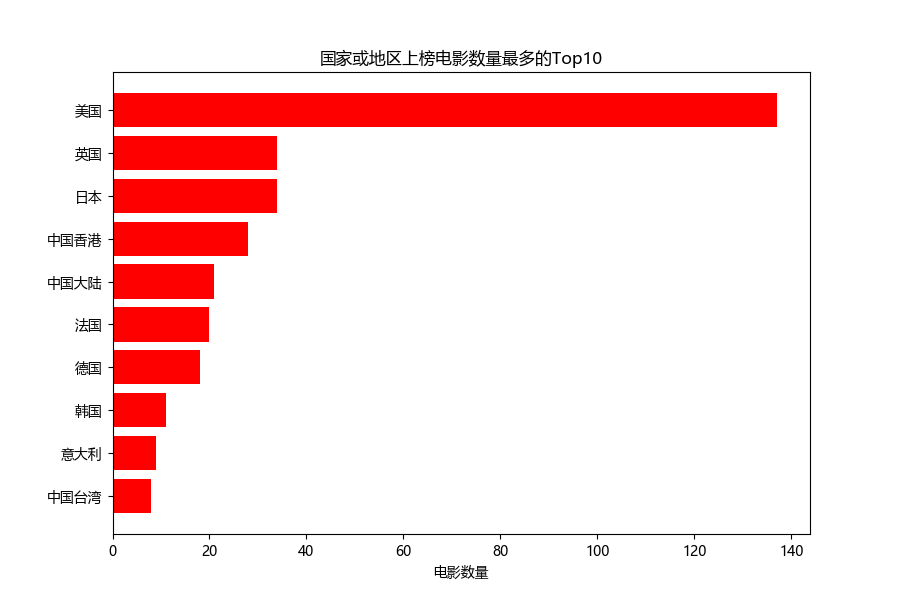5. 豆瓣电影Top250-评价人数Top10

import pandas as pd
from matplotlib import pyplot as plt

df = pd.read_excel('movie.xlsx')
name = list(df['电影名'])
ranting_num = list(df['评价人数'])
# (电影名, 评价人数)
info = [(m, int(n.split('人'))) for m, n in list(zip(name, ranting_num))]
# 按评价人数排序
info.sort(key=lambda x: x)
# print(info)

name = [x for x in info[-10:]]
ranting_num = [x for x in info[-10:]]

plt.figure(figsize=(12, 6), dpi=100)
# 设置字体
plt.rcParams['font.sans-serif'] = ['Microsoft YaHei']

# 绘制横着的条形图
plt.barh(name, ranting_num, color='cyan', height=0.4)

# 添加描述信息
plt.xlabel('评价人数')
plt.title('豆瓣电影Top250-评价人数Top10')

plt.savefig('test5.PNG')
plt.show()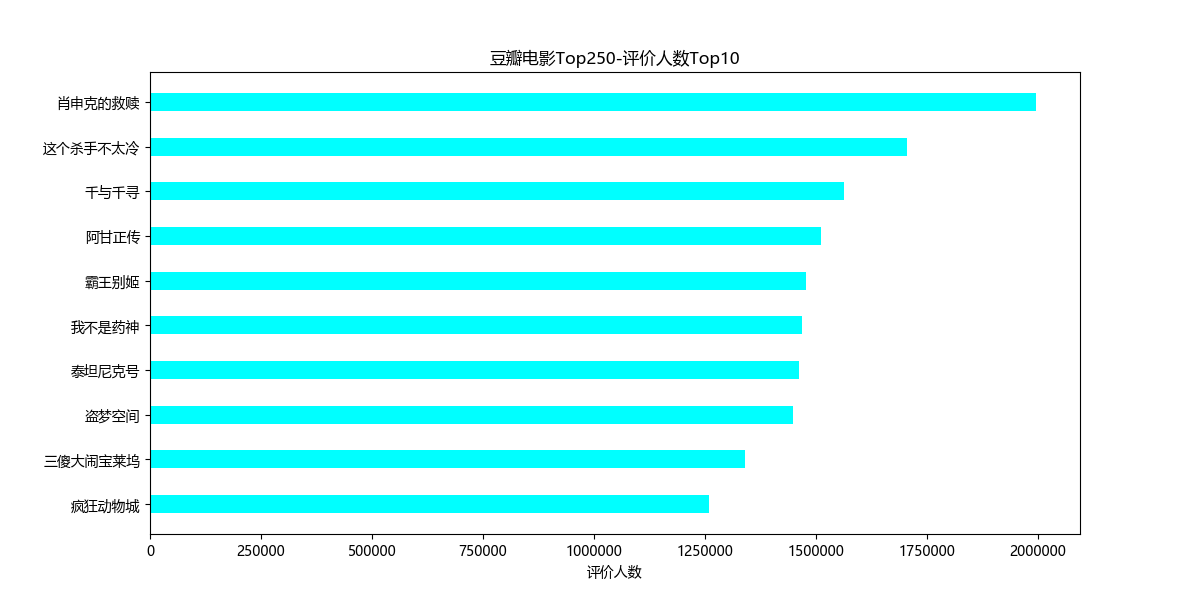6. 对肖申克的救赎的部分评论进行文本分词并绘制词云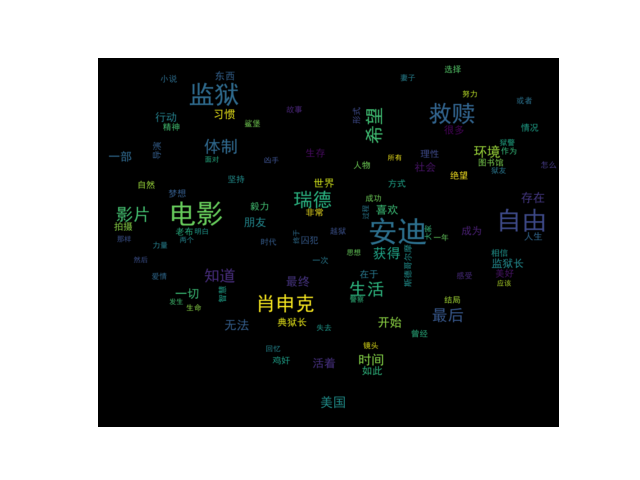7. 补充说明

本项目仅用于技术交流和学习
欢迎提出改进意见，以期共同进步。

展开全文数据分析 大数据 数据挖掘
• 向AI转型的程序员都关注了这个号????????????机器学习AI算法工程 公众号：datayxpython数据可视化编程实战一本使用Python实现数据可视化编程的实战指南书籍。介...
• 大家好，今天让我们看一下使用Python进行数据可视化的主要库以及可以使用它们完成的所有类型的图表。我们还将看到建议在每种情况下使用哪个库以及每个库的独特功能。 我们将从最基本的可视化开始，直接查看数据，...编程语言
• 点击上方“Python爬虫与数据挖掘”，进行关注回复“书籍”即可获赠Python从入门到进阶共10本电子书今日鸡汤借问酒家何处有，牧童遥指杏花村。大家好，我是宝器！啰里八嗦开场就不多写了...
• 1 准备11.1 开发环境搭建21.1.1 在Ubuntu系统下搭建Python集成开发环境21.1.2 在Windows系统下搭建Python集成开发环境131.1.3 三种安装第三方库的方法161.2 Python基础语法介绍191.2.1 if__name__=="...
• （2）专注于Python数据分析与可视化操作中实际用到的技术。相比大而全的书籍资料，本书能让读者尽快上手，开始项目开发。（3）书中的“新手问答”和“小试牛刀”栏目能让读者巩固知识，举一反三，学以致用。《Python...
• 那么针对这三类人，我给大家提供一个好的学习平台，免费领取视频教程，电子书籍，以及课程的源代码！ QQ群：101677771 主要的步骤如下： 1.从.xlsx文件中读出制图所需要的的数据 2.使用matplotlib库绘图并保存 ...可视化
• 点击上方“Python爬虫与数据挖掘”，进行关注回复“书籍”即可获赠Python从入门到进阶共10本电子书今日鸡汤时穷节乃现，一一垂丹青。本文含5062字，19图表截屏建议阅读 1...可视化 编程语言 数据分析
• （2）专注于Python数据分析与可视化操作中实际用到的技术。相比大而全的书籍资料，本书能让读者尽快上手，开始项目开发。（3）书中的"新手问答”和"小试牛刀”栏目能让读者巩固知识，举一反三，学以致用。...
• 本资源包含两本：python数据可视化编程实战中文版和数据可视化手册英文版。
• Python提供了很多模块用于数据可视化，其中matplotlib、pygal等模块。我参考网上热门书籍Python编程从入门到实战》，在测试与学习过程中遇到的些许问题加以解决，才写下这一项目实战的心得，对于Python基础部分就...
• 点击上方“Python爬虫与数据挖掘”，进行关注回复“书籍”即可获赠Python从入门到进阶共10本电子书今日鸡汤我行殊未已，何日复归来。导读：制作提供信息的可视化(有时称为绘图)是数据...可视化 数据分析 java
• ospybook库来自《Python地理数据处理》书籍，用于地理数据的简单显示 【书】《Python地理数据处理》[美] Chris Garrard 著 2017年7月 【文件夹介绍】本文件夹为该书的...文章目录ospybook介绍——Python可视化地...
• 如同艺术家们用绘画让人们更贴切的感知世界，数据可视化也能让人们更直观的传递数据所要表达的信息。你知道Python脚本可视化有多好看么？就像下图这样，是不是感觉十分高端大气上档次： 很多人学习python，不知道从...编程语言
• 制作词云图一.词云图介绍二.wordcloud方法二....词云图的应用相当广泛，诸如电影-视频-微博-网易云-淘宝-知乎等评论分析、书籍-报告-商品信息-疫情舆论等文本分析，使用词云图能使数据信息的表达一目了然。
• 这是《Python数据挖掘课程》系列文章，也是我这学期上课的部分内容。本文主要讲述鸢尾花数据集的各种分析，包括可视化分析、线性回归分析、决策树分析等，通常一个数据集是可以用于多种分析的，希望这篇文章对大家...回归分析 决策树
• python可视化Task01 我学绘图是从R语言起步的，R语言在绘图方面有很成熟的ggplot2包和衍生出的成熟生态，市面上也有很多不错的书籍资料，对ggplot2图层叠加的绘图思想讲解也比较透彻。但后面感觉到R在数据处理方面的...matplotlib
• 图形可视化是展示数据的一个非常好的手段，好的图表自己会说话。毋庸多言，在Python的世界里，matplotlib是最著名的绘图库，它支持几乎所有的2D绘图和部分3D绘图，被...《Python数据可视化编程实战（第2版）》则是一...
• 点击上方“Python爬虫与数据挖掘”，进行关注回复“书籍”即可获赠Python从入门到进阶共10本电子书今日鸡汤入我相思门，知我相思苦。专栏作者：zch，经管专业研一在读，Python数...可视化 数据分析 nagios csv
• 从发行版的安装开始，这本书将科学计算及可视化的常见函数库，如numpy、scipy、sympy、matplotlib、traits、tvtk、mayavi、opencv等等，都进行了较为详细地介绍。由于涉及面太广，可能对于单个函数库来说还不够深入...
• 电子书籍、项目源码分享。一起交流学习，一起进步！ 前言 本文的文字及图片来源于网络,仅供学习、交流使用,不具有任何商业用途,如有问题请及时联系我们以作处理。 基本开发环境 Python 3.6 Pycharm 相关模块...
• 数据提取 在我之前的文章Scrapy自动爬取商品数据爬虫里实现了爬虫爬取商品网站搜索关键词为python书籍商品，爬取到了60多页...接下来对爬取到的商品数据作预处理及可视化分析，使用工具为Anaconda的Jupyter n......

# python数据可视化书籍python 订阅